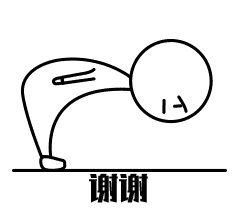# PixiJs学前篇（四）：Canvas进阶【动画篇】🔥🔥

## theme: smartblue highlight: a11y-dark# 前言

PixiJs学前篇（一）：Canvas基础【绘制篇】

PixiJs学前篇（二）：Canvas基础【图形篇】

PixiJs学前篇（三）：Canvas基础【文字篇】

# 动画

## 几何变换

### 移动

``````<!DOCTYPE html>
<html lang="en">
<meta charset="UTF-8">
<meta http-equiv="X-UA-Compatible" content="IE=edge">
<meta name="viewport" content="width=device-width, initial-scale=1.0">
<title>canvas - 动画</title>
<body>
<canvas
id="canvas"
width="550"
height="500"
当前浏览器不支持canvas元素，请升级或更换浏览器！
</canvas>
<script>
const canvas = document.getElementById('canvas'); // 获取Canvas
const ctx = canvas.getContext('2d'); // 获取绘制上下文
ctx.fillStyle="#ff0000"

// 向x轴和y轴平移200像素
ctx.translate(200, 200);
// 在（0,0）坐标点绘制一个宽：200，高：100的矩形
ctx.fillRect(0, 0, 200, 100)

</script>
</body>
</html>
``````### 旋转

``````<!DOCTYPE html>
<html lang="en">
<meta charset="UTF-8">
<meta http-equiv="X-UA-Compatible" content="IE=edge">
<meta name="viewport" content="width=device-width, initial-scale=1.0">
<title>canvas - 动画</title>
<body>
<canvas
id="canvas"
width="550"
height="500"
当前浏览器不支持canvas元素，请升级或更换浏览器！
</canvas>
<script>
const canvas = document.getElementById('canvas'); // 获取Canvas
const ctx = canvas.getContext('2d'); // 获取绘制上下文
for (let i = 0; i < 9; i++) {
ctx.fillStyle=`#\${i}\${i}\${i}`
// 旋转弧度设置，角度和弧度的转换公式：1° = Math.PI / 180
ctx.rotate(i * 2 * Math.PI / 180);
// 在（0,0）坐标点绘制一个宽：200，高：100的矩形
ctx.fillRect(100, 0, 200, 100)
}
</script>
</body>
</html>

``````### 缩放

``````<!DOCTYPE html>
<html lang="en">
<meta charset="UTF-8">
<meta http-equiv="X-UA-Compatible" content="IE=edge">
<meta name="viewport" content="width=device-width, initial-scale=1.0">
<title>canvas - 动画</title>
<body>
<canvas
id="canvas"
width="550"
height="500"
当前浏览器不支持canvas元素，请升级或更换浏览器！
</canvas>
<script>
const canvas = document.getElementById('canvas'); // 获取Canvas
const ctx = canvas.getContext('2d'); // 获取绘制上下文

for (let i = 0; i < 9; i++) {
ctx.fillStyle=`#\${i}\${i}\${i}`
ctx.beginPath()
ctx.scale(2 / i, 2 / i);
// 绘制圆
ctx.arc(250, 250, 50, 0, 360 * Math.PI/180);
ctx.fill();
}
</script>
</body>
</html>

``````## 状态的保存和恢复

• 应用的裁切路径（clipping path）

``````<!DOCTYPE html>
<html lang="en">
<meta charset="UTF-8">
<meta http-equiv="X-UA-Compatible" content="IE=edge">
<meta name="viewport" content="width=device-width, initial-scale=1.0">
<title>canvas - 动画</title>
<body>
<canvas
id="canvas"
width="550"
height="500"
当前浏览器不支持canvas元素，请升级或更换浏览器！
</canvas>
<script>
const canvas = document.getElementById('canvas'); // 获取Canvas
const ctx = canvas.getContext('2d'); // 获取绘制上下文

ctx.fillStyle = "gray";
ctx.fillRect(10, 10, 200, 100);
// 保存状态
ctx.save();
ctx.fillStyle = "orange";
ctx.fillRect(10, 150, 200, 100);
// 恢复上次保存的状态
ctx.restore();
ctx.fillRect(10, 300, 200, 200);

</script>
</body>
</html>
``````## 动画

• setInterval(function, delay) ：定时器，当设定好间隔时间后，function 会定期执行。
• setTimeout(function, delay)：延时器，在设定好的时间之后执行函数
• requestAnimationFrame(callback)：告诉浏览器你希望执行一个动画，并在重绘之前，请求浏览器执行一个特定的函数来更新动画。

### setInterval、setTimeout

``````<!DOCTYPE html>
<html lang="en">
<meta charset="UTF-8">
<meta http-equiv="X-UA-Compatible" content="IE=edge">
<meta name="viewport" content="width=device-width, initial-scale=1.0">
<title>canvas - 动画</title>
<body>
<canvas
id="canvas"
width="550"
height="500"
当前浏览器不支持canvas元素，请升级或更换浏览器！
</canvas>
<script>
const canvas = document.getElementById('canvas'); // 获取Canvas
const ctx = canvas.getContext('2d'); // 获取绘制上下文
ctx.fillStyle = "#ccc";
let num = 0
setInterval(()=>{
num += 1
if(num <= 400) {
ctx.fillRect(num, 0, 100, 100);
}
})
</script>
</body>
</html>

``````### 画布清空

• x为要清除的矩形区域左上角的x坐标，
• y为要清除的矩形区域左上角的y坐标
• width为要清除的矩形区域的宽度
• height为要清除的矩形区域的高度

``````<!DOCTYPE html>
<html lang="en">
<meta charset="UTF-8">
<meta http-equiv="X-UA-Compatible" content="IE=edge">
<meta name="viewport" content="width=device-width, initial-scale=1.0">
<title>canvas - 动画</title>
<body>
<canvas
id="canvas"
width="550"
height="500"
当前浏览器不支持canvas元素，请升级或更换浏览器！
</canvas>
<script>
const canvas = document.getElementById('canvas'); // 获取Canvas
const ctx = canvas.getContext('2d'); // 获取绘制上下文
ctx.fillStyle = "#ccc";
const width = canvas.width
const height = canvas.height
let num = 0
setInterval(()=>{
num += 1
if(num <= 400) {
ctx.clearRect(0, 0, width, height)
ctx.fillRect(num, 0, 100, 100);
}
})
</script>
</body>
</html>
``````nice！如图我们就把我们想要的效果实现了，

### requestAnimationFrame

`requestAnimationFrame()`方法的整体性能要比`setInterval(）`方法好很多，打个比方，当我们用`setInterval(）`方法来做动画，我们需要设置一下多长时间执行一次`setInterval(）`方法里面的代码块，而这个时间我们只要设定了，那么就会强行这个时间执行，而如果我们的浏览器显示频率和`setInterval(）`方法执行的绘制请求不一致，就会导致一些帧率消息，从而造成卡顿的效果。因此使用`requestAnimationFrame()`方法做动画会更加平缓且有效率。

``````function callbackFn() {
// 放入需要执行的代码块
requestAnimationFrame(callbackFn);
}
requestAnimationFrame(callbackFn);

``````

``````<!DOCTYPE html>
<html lang="en">
<meta charset="UTF-8">
<meta http-equiv="X-UA-Compatible" content="IE=edge">
<meta name="viewport" content="width=device-width, initial-scale=1.0">
<title>canvas - 动画</title>
<body>
<canvas
id="canvas"
width="550"
height="500"
当前浏览器不支持canvas元素，请升级或更换浏览器！
</canvas>
<script>
// 获取Canvas
const canvas = document.getElementById('canvas');
// 获取绘制上下文
const ctx = canvas.getContext('2d');
// globalCompositeOperation 属性设置或返回如何将一个源（新的）图像绘制到目标（已有的）的图像上。
// 这里主要是为了让飞机压在运行轨迹上
ctx.globalCompositeOperation = 'destination-over';
const width = canvas.width
const height = canvas.height
let num = 0
ctx.strokeStyle = "#ccc"
const img = new Image()
img.src="../images/plane.png"
requestAnimationFrame(planeRun);
}
function planeRun(){
// 清空画布
ctx.clearRect(0, 0, width, height)

// 保存画布状态
ctx.save();

// 把原心移到画布中间
ctx.translate(250, 250);

// 绘制飞机和飞机动画
num += 0.01
ctx.rotate(-num);
ctx.translate(0, 200);
ctx.drawImage(img, -20, -25, 40, 40);

// 恢复状态
ctx.restore();

// 飞机运行的轨迹
ctx.beginPath();
ctx.arc(250, 250, 200, 0, Math.PI * 2, false);
ctx.stroke();

// 执行完以后继续调用
requestAnimationFrame(planeRun);
}
</script>
</body>
</html>

``````## transform`矩阵`如果展开来说内容太多，这里咱们就简单说一下`transform`

`transform`不仅能实现`移动``旋转``缩放`，还能实现`斜切`

### 语法：

`transform(a, b, c, d, e, f)`

• a：水平缩放，不缩放为1
• b：水平倾斜，不倾斜为0
• c：垂直倾斜，不倾斜为0
• d：垂直缩放，不缩放为1
• e：水平移动，不移动为0
• f：垂直移动，不移动为0

### 移动

``````<!DOCTYPE html>
<html lang="en">
<meta charset="UTF-8">
<meta http-equiv="X-UA-Compatible" content="IE=edge">
<meta name="viewport" content="width=device-width, initial-scale=1.0">
<title>canvas - transform</title>
<body>
<canvas
id="canvas"
width="550"
height="500"
当前浏览器不支持canvas元素，请升级或更换浏览器！
</canvas>
<script>
// 获取Canvas
const canvas = document.getElementById('canvas');
// 获取绘制上下文
const ctx = canvas.getContext('2d');
// 填充颜色
ctx.fillStyle="orange"

// x轴和y轴都移动了100
ctx.transform(1, 0, 0, 1, 100, 100);

ctx.fillRect(0, 0, 200, 200);

</script>
</body>
</html>
``````### 缩放

``````<!DOCTYPE html>
<html lang="en">
<meta charset="UTF-8">
<meta http-equiv="X-UA-Compatible" content="IE=edge">
<meta name="viewport" content="width=device-width, initial-scale=1.0">
<title>canvas - transform</title>
<body>
<canvas
id="canvas"
width="550"
height="500"
当前浏览器不支持canvas元素，请升级或更换浏览器！
</canvas>
<script>
// 获取Canvas
const canvas = document.getElementById('canvas');
// 获取绘制上下文
const ctx = canvas.getContext('2d');
// 填充颜色
ctx.fillStyle="orange"

// x轴和y轴都放大1.5倍
ctx.transform(1.5, 0, 0, 1.5, 0, 0);
ctx.fillRect(0, 0, 200, 200);

</script>
</body>
</html>
``````### 斜切

``````<!DOCTYPE html>
<html lang="en">
<meta charset="UTF-8">
<meta http-equiv="X-UA-Compatible" content="IE=edge">
<meta name="viewport" content="width=device-width, initial-scale=1.0">
<title>canvas - transform</title>
<body>
<canvas
id="canvas"
width="550"
height="500"
当前浏览器不支持canvas元素，请升级或更换浏览器！
</canvas>
<script>
// 获取Canvas
const canvas = document.getElementById('canvas');
// 获取绘制上下文
const ctx = canvas.getContext('2d');
// 填充颜色
ctx.fillStyle="orange"

//Y轴逐渐拉伸，得到上图右边状态
ctx.transform(1, Math.PI/20, 0, 1, 0, 0);

ctx.fillRect(150, 100, 200, 200);

</script>
</body>
</html>
``````### 旋转

``````<!DOCTYPE html>
<html lang="en">
<meta charset="UTF-8">
<meta http-equiv="X-UA-Compatible" content="IE=edge">
<meta name="viewport" content="width=device-width, initial-scale=1.0">
<title>canvas - transform</title>
<body>
<canvas
id="canvas"
width="550"
height="500"
当前浏览器不支持canvas元素，请升级或更换浏览器！
</canvas>
<script>
// 获取Canvas
const canvas = document.getElementById('canvas');
// 获取绘制上下文
const ctx = canvas.getContext('2d');
// 填充颜色
ctx.fillStyle="orange"

// 旋转30度
const deg = Math.PI/180;
const c3 = Math.cos(30*deg)
const s3 = Math.sin(30*deg)
ctx.transform(c3, s3, -s3, c3, 0, 0)
ctx.fillRect(0, 0, 200, 200);
</script>
</body>
</html>
``````